Successfully reported this slideshow.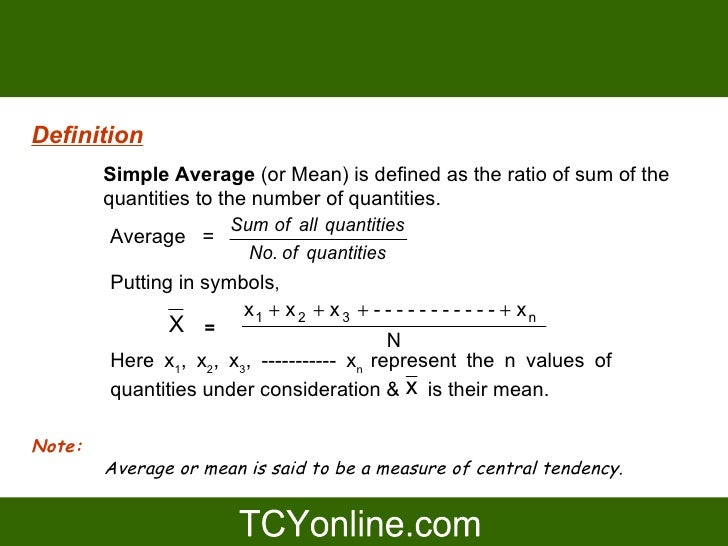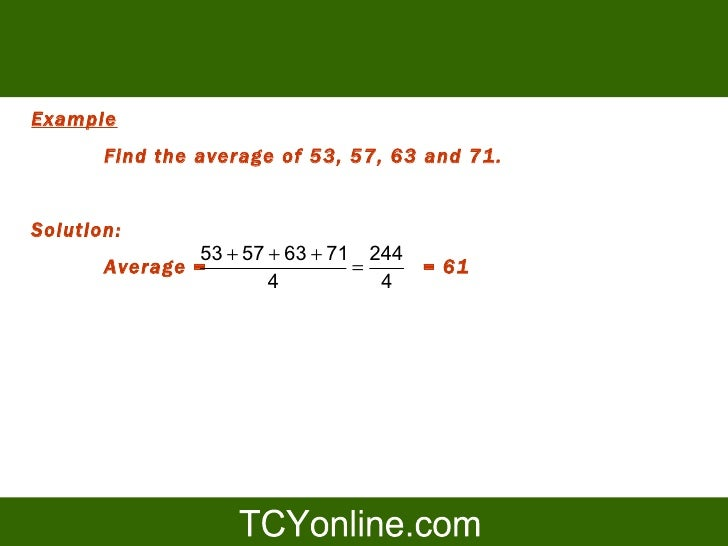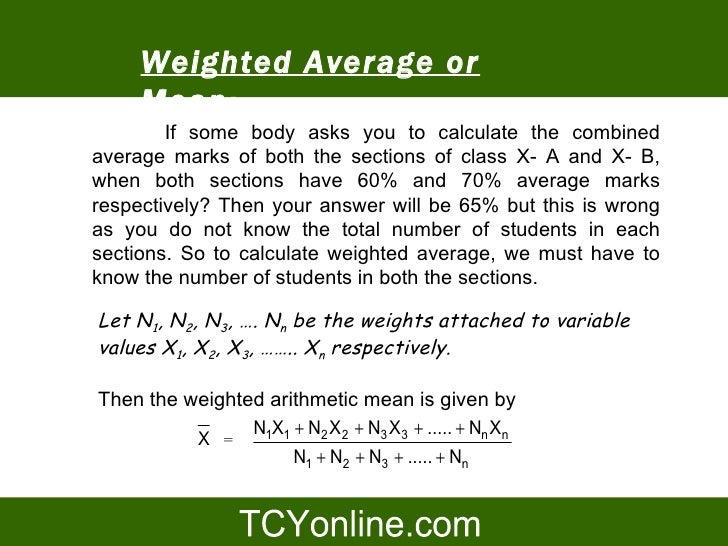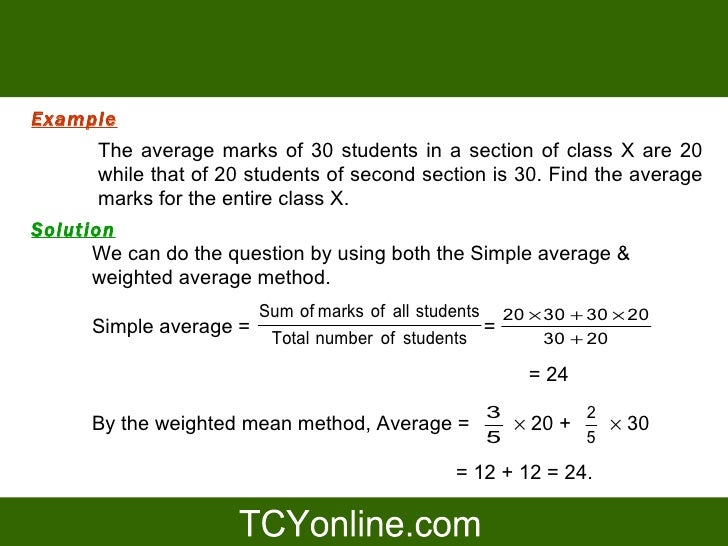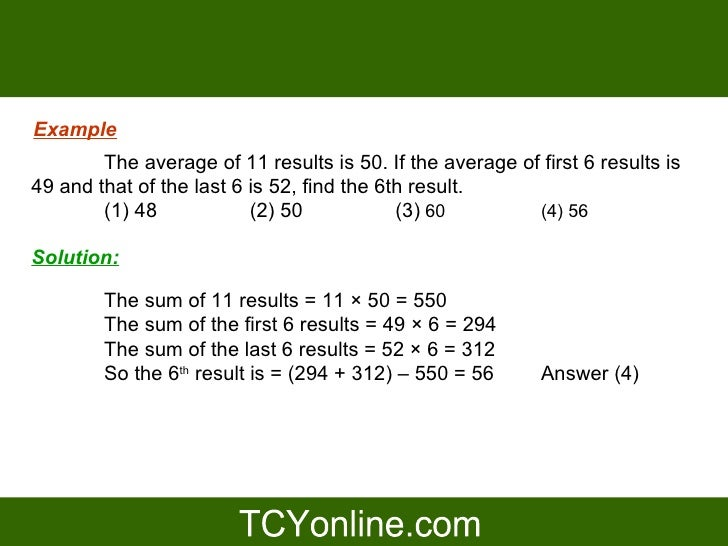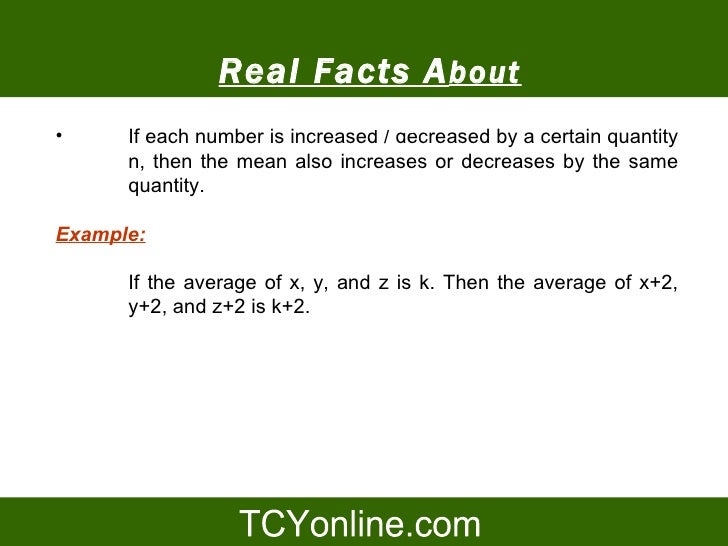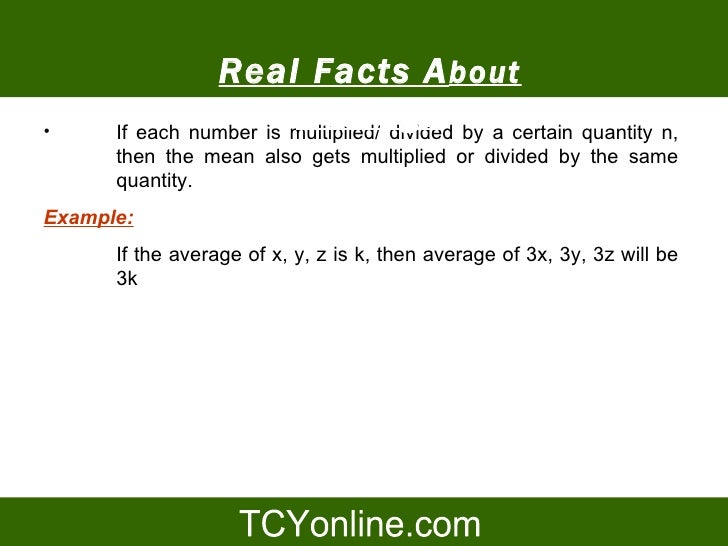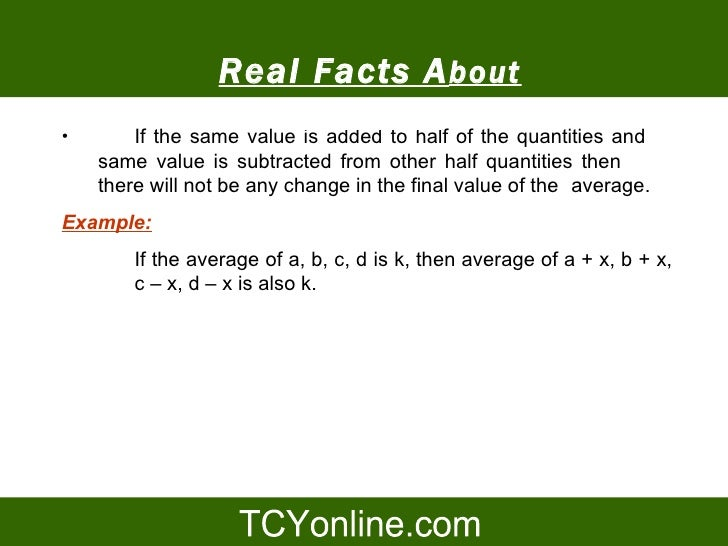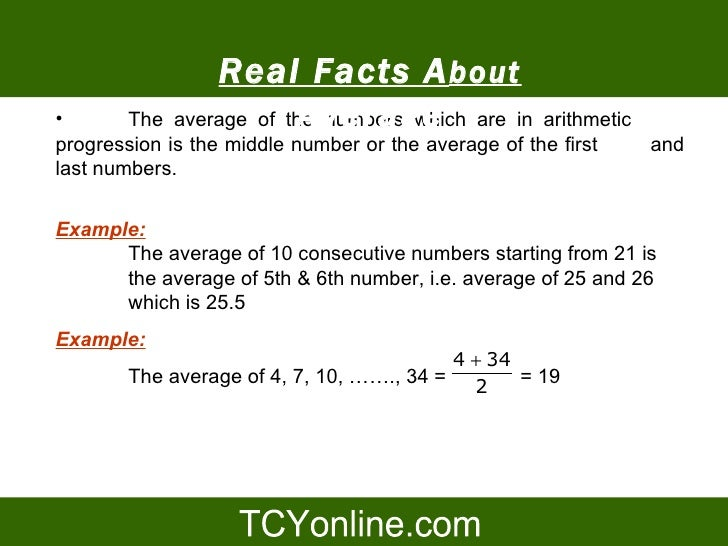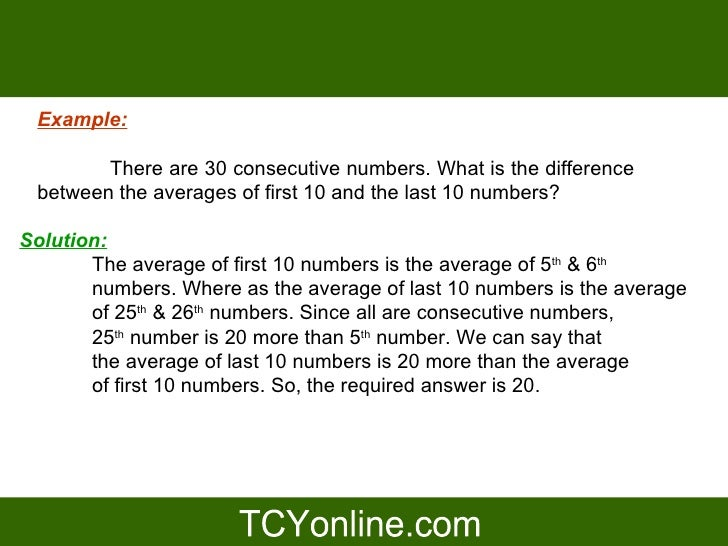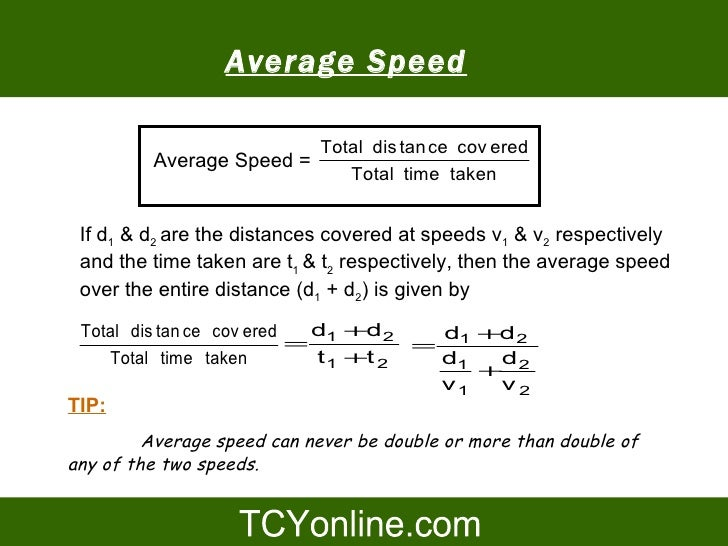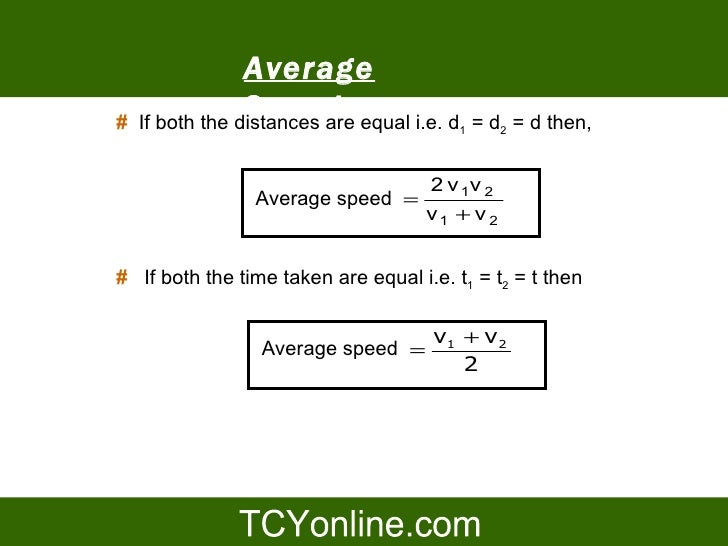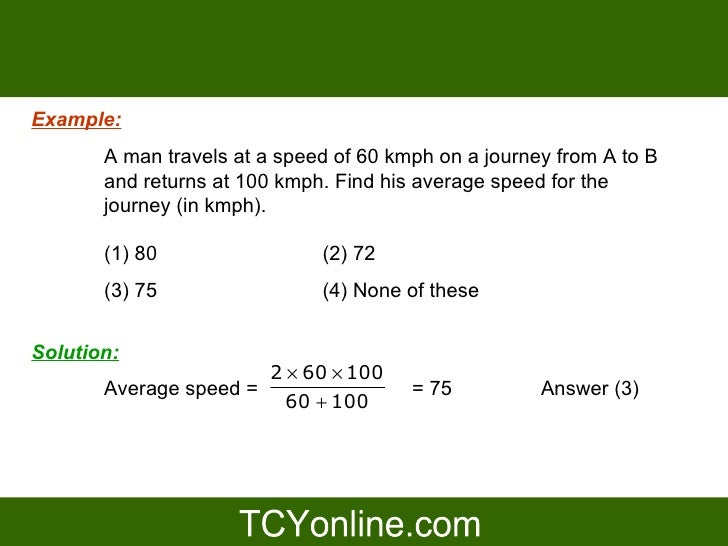Upcoming SlideShare
×
• Full Name
Comment goes here.

Are you sure you want to Yes No• Be the first to comment

### Ppt On Average CAT quant 2009

1. 1. Average
2. 2. Definition Simple Average (or Mean) is defined as the ratio of sum of the quantities to the number of quantities. Sum of all quantities Average = No. of quantities Putting in symbols, x1 + x 2 + x 3 + - - - - - - - - - - - + x n X = N Here x1, x2, x3, ----------- xn represent the n values of quantities under consideration & x is their mean. Note: Average or mean is said to be a measure of central tendency.
3. 3. Example Find the average of 53, 57, 63 and 71. Solution: 53 + 57 + 63 + 71 244 Average = = = 61 4 4
4. 4. Weighted Average or Mean: If some body asks you to calculate the combined average marks of both the sections of class X- A and X- B, when both sections have 60% and 70% average marks respectively? Then your answer will be 65% but this is wrong as you do not know the total number of students in each sections. So to calculate weighted average, we must have to know the number of students in both the sections. Let N1, N2, N3, …. Nn be the weights attached to variable values X1, X2, X3, …….. Xn respectively. Then the weighted arithmetic mean is given by N1X1 + N2 X2 + N3 X3 + ..... + Nn Xn X = N1 + N2 + N3 + ..... + Nn
5. 5. Example The average marks of 30 students in a section of class X are 20 while that of 20 students of second section is 30. Find the average marks for the entire class X. Solution We can do the question by using both the Simple average & weighted average method. Sum of marks of all students 20 × 30 + 30 × 20 Simple average = = Total number of students 30 + 20 = 24 3 2 By the weighted mean method, Average = × 20 + × 30 5 5 = 12 + 12 = 24.
6. 6. Example The average of 11 results is 50. If the average of first 6 results is 49 and that of the last 6 is 52, find the 6th result. (1) 48 (2) 50 (3) 60 (4) 56 Solution: The sum of 11 results = 11 × 50 = 550 The sum of the first 6 results = 49 × 6 = 294 The sum of the last 6 results = 52 × 6 = 312 So the 6th result is = (294 + 312) – 550 = 56 Answer (4)
7. 7. Real Facts A bout • Average If each number is increased / decreased by a certain quantity n, then the mean also increases or decreases by the same quantity. Example: If the average of x, y, and z is k. Then the average of x+2, y+2, and z+2 is k+2.
8. 8. Real Facts A bout • Average If each number is multiplied/ divided by a certain quantity n, then the mean also gets multiplied or divided by the same quantity. Example: If the average of x, y, z is k, then average of 3x, 3y, 3z will be 3k
9. 9. Real Facts A bout • Average of the quantities and If the same value is added to half same value is subtracted from other half quantities then there will not be any change in the final value of the average. Example: If the average of a, b, c, d is k, then average of a + x, b + x, c – x, d – x is also k.
10. 10. Real Facts A bout • Average The average of the numbers which are in arithmetic progression is the middle number or the average of the first and last numbers. Example: The average of 10 consecutive numbers starting from 21 is the average of 5th & 6th number, i.e. average of 25 and 26 which is 25.5 Example: 4 + 34 The average of 4, 7, 10, ……., 34 = = 19 2
11. 11. Example: There are 30 consecutive numbers. What is the difference between the averages of first 10 and the last 10 numbers? Solution: The average of first 10 numbers is the average of 5th & 6th numbers. Where as the average of last 10 numbers is the average of 25th & 26th numbers. Since all are consecutive numbers, 25th number is 20 more than 5th number. We can say that the average of last 10 numbers is 20 more than the average of first 10 numbers. So, the required answer is 20.
12. 12. Average Speed Total dis tan ce cov ered Average Speed = Total time taken If d1 & d2 are the distances covered at speeds v1 & v2 respectively and the time taken are t1 & t2 respectively, then the average speed over the entire distance (d1 + d2) is given by Total dis tan ce cov ered d +d2 d +d2 = 1 = 1 Total time taken t 1 +t 2 d1 d + 2 v1 v2 TIP: Average speed can never be double or more than double of any of the two speeds.
13. 13. Average # Speed If both the distances are equal i.e. d = d2 = d then, 1 2 v 1v 2 Average speed = v1 + v 2 # If both the time taken are equal i.e. t1 = t2 = t then v1 + v2 Average speed = 2
14. 14. Example: A man travels at a speed of 60 kmph on a journey from A to B and returns at 100 kmph. Find his average speed for the journey (in kmph). (1) 80 (2) 72 (3) 75 (4) None of these Solution: 2 × 60 × 100 Average speed = = 75 Answer (3) 60 + 100
15. 15. Thank you !!

### Be the first to comment

•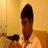#### elangonit

Oct. 10, 2009
•#### msnarayanannarayanan

Sep. 20, 2015
•Jan. 9, 2020

Total views

5,452

On Slideshare

0

From embeds

0

Number of embeds

20

307

Shares

0